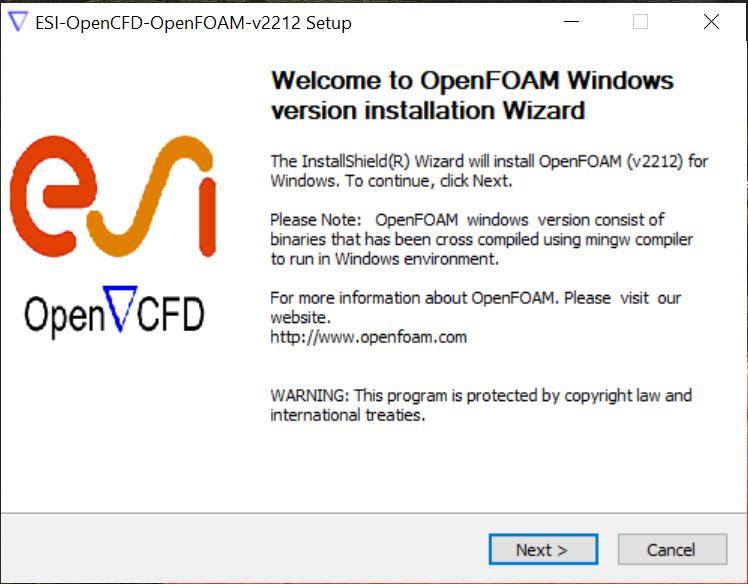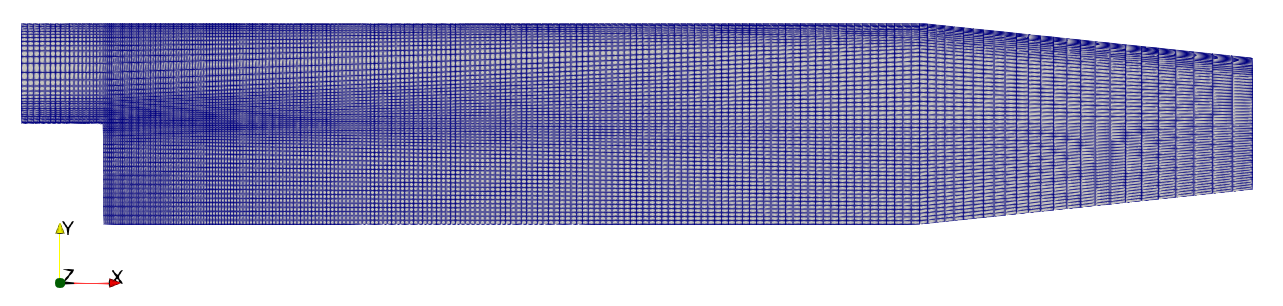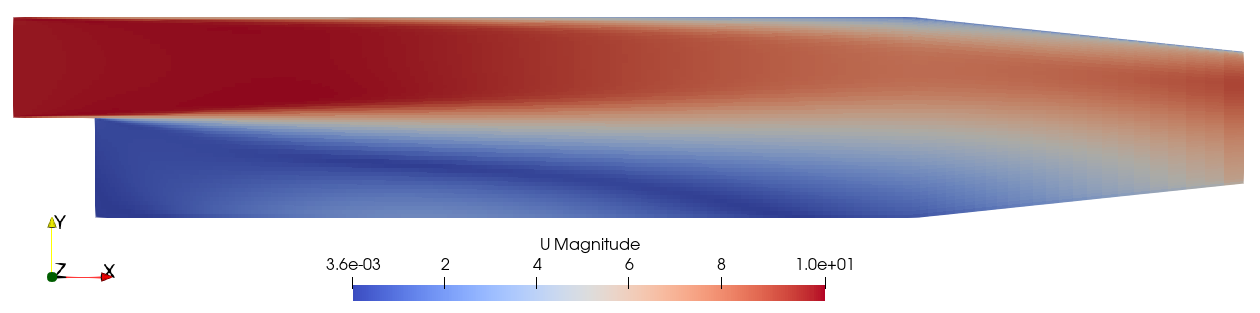Welcome to the OpenFOAM® quickstart guide!

This guide provides a simplified step-by-step overview to help you quickly get started with the installation, setup, and basic usage of OpenFOAM®, a powerful free/open-source computational fluid dynamics (CFD) software.

## OpenFOAM® Installation🔗

OpenFOAM® is easily accessible on a wide range of operating systems, including Linux distributions, Microsoft Windows, and macOS. You can conveniently obtain OpenFOAM® in the form of precompiled packages or as source code that you can build yourself.

In this guide, we will install the precompiled package of the latest OpenFOAM® version on Microsoft Windows 10 Enterprise operating system.

Download the package of the latest OpenFOAM® version by simply clicking the link below (Please ensure that you have at least 300MB of available storage space on your hard disk):

The package is an executable. Run this executable to open the installation wizard, and simply follow the wizard’s installation instructions specific to your system. A typical view of the wizard is shown below (Please ensure that you have enough available storage space on your hard disk):You may also need to install Microsoft MPI (MS-MPI). Similary, download the following package (i.e. msmpisetup.exe), and follow its installation wizard:

Upon completion of the above steps, an icon will be created on your desktop. Double-click this icon to launch a terminal window. Running this icon will load the OpenFOAM® environment. Verify the contents of OpenFOAM® in a terminal as follows ($ is the command prompt, indicating that the terminal is ready to accept a command.): $ ls -p
OpenFOAM/

$cd$WM_PROJECT_DIR

$ls -p bin/ COPYING etc/ etc-mingw META-INFO platforms/ README.md ThirdParty/ tutorials/  ## Problem Setup🔗 The problem setup is derived from the experiment conducted by Pitz and Daily (1983). The setup involves a steady simulation of a non-reacting, single-phase, Newtonian, incompressible fluid flow, which comprises a range of intricate turbulence phenomena such as flow detachment/reattachment and formation of recirculation zones. You can browse the problem setup: $ cd $FOAM_TUTORIALS/incompressible/simpleFoam/pitzDaily/$ ls -p
0/  constant/  system/

$ls -p system/ blockMeshDict controlDict fvSchemes fvSolution streamlines$ ls -p constant/
transportProperties  turbulenceProperties

$ls -p 0/ epsilon k p U  In OpenFOAM®, simulation settings are customised using text files. Each parameter in these files follows a straightforward key-value pair format. However, it is important to note that each file has its own unique set of mandatory and optional keywords. The location and content of these files vary depending on the chosen solver application, simulation setup, and the specific aspects you wish to modify. Therefore, it is necessary to familiarize yourself with the structure and significance of these keywords in relevant files. ## Pre-process🔗 Pre-processing involves the typical preparation steps of a CFD problem setup. To carry out these steps, it is necessary to be familiar first with the typical directory structure of an OpenFOAM® setup and the meaning of these directories. An OpenFOAM® simulation generally consists of the following three directories: • 0: (zero directory) contains field initial/boundary conditions at timestep (or iteration) zero. Note that fields are typically named according to their mathematical representation, e.g. u for velocity, p for pressure, T for temperature etc. • constant: contains files that define the geometric and physical properties of the simulation. • system: contains files that define the settings of the simulation applications and tools. ### Physical domain🔗 In OpenFOAM®, there are multiple ways to provide the physical domain specifications for a simulation. These include: • Geometry import: OpenFOAM® supports the import of geometry from external sources in formats such as STL, STEP, or IGES, to name a few. • Geometry definition: OpenFOAM® allows manual definition of the physical domain within itself. • Mesh generation: OpenFOAM® provides native meshing tools including blockMesh, snappyHexMesh, and mesh converters to generate meshes that represent the physical domain. In this example, we generate the physical domain alongside the mesh using the blockMesh utility. The physical domain includes a narrow inlet passage, a backward-facing step, and a converging section, as shown in the side view below:### Physical properties🔗 In this example, we consider a simple non-reacting, single-phase, Newtonian, incompressible fluid flow, with the following physical properties: Metrics Values Fluid kinematic viscosity, $$\nu$$ $$1e\text{-}5\,[\mathrm{m}^2\text{/}\mathrm{s}]$$ In OpenFOAM®, the type of fluid and the value of the fluid kinematic viscosity are set using the transportProperties file located in the constant directory. $ cat constant/transportProperties

[...]

transportModel  Newtonian;
nu              1e-05;

[...]


### Spatial domain discretisation🔗

In this example, we generate the spatial domain discretisation, also known as the mesh, using the blockMesh utility configured by the blockMeshDict file in the system directory.

You can skim through the content of this file via (for further information, please see blockMesh):

$cat system/blockMeshDict  The side view of the generated mesh is illustrated below:In OpenFOAM®, the spatial domain discretization is typically performed using one of three methods, depending on the complexity of the physical domain and the desired mesh characteristics: • blockMesh: built-in mesh generation tool in OpenFOAM® providing a simple and intuitive way to generate structured meshes for simple geometries. • snappyHexMesh: powerful built-in mesh generation tool in OpenFOAM® used for generating high-quality, unstructured meshes. It can handle complex geometries and supports various meshing strategies, such as refinement regions and boundary layer additions. • Mesh conversion: OpenFOAM® supports the import of meshes generated by various external mesh generators using tools like fluentMeshToFoam. ### Temporal domain discretisation🔗 In this example, the temporal domain discretization, i.e. the division of the simulation time into discrete steps, is steady state. In OpenFOAM®, the temporal domain discretization is set in the controlDict file located in the system directory. The following key-value pairs in the controlDict file allow users to configure the temporal behaviour of the simulation: $ cat system/controlDict

[...]

startFrom       startTime;
startTime       0;
stopAt          endTime;
endTime         2000;
deltaT          1;

[...]


OpenFOAM® provides several methods to handle the temporal discretization, allowing users to control the time advancement and accuracy of their simulations. Here are the main methods for generating the temporal domain discretization in OpenFOAM®:

• Explicit time stepping
• Implicit time stepping

### Equation discretisation🔗

In OpenFOAM®, the equation discretization refers to the numerical approximation of the governing equations that describe fluid flow or other physical phenomena. OpenFOAM® provides a wide range of discretization schemes that can be used to discretize the equations and solve them numerically.

The selection of discretization schemes is usually defined for each equation field and equation type in the fvSchemes file found in the system directory.

Within the fvSchemes file, you will find configurations for discretization schemes applied to gradient, divergence, Laplacian, and other terms in the governing equations.

For instance, you can examine the settings for time and gradient schemes within the fvSchemes file, as shown below:

$cat system/fvSchemes [...] ddtSchemes { default steadyState; } gradSchemes { default Gauss linear; } [...]  ### Initial/boundary conditions🔗 In this example, the initial and boundary conditions of the governing equations at geometric boundaries are set as follows: Property Inlet Outlet Walls Internal $$\text{Velocity}, \u \, [\text{m/s}]$$ $$(10, 0, 0)$$ $$\grad \u = \tensor{0}$$ $$(0, 0, 0)$$ $$(0, 0, 0)$$ $$\text{Pressure}, p \, [\mathrm{m}^2\text{/}\mathrm{s}^2]$$ $$\grad p = \vec{0}$$ $$0$$ $$\grad p = \vec{0}$$ $$0$$ $$\text{Turbulent kinetic energy}, k \, [\mathrm{m}^2\text{/}\mathrm{s}^2]$$ $$0.375$$ $$\grad k = \vec{0}$$ $$\text{wall function}$$ $$0.375$$ $$\text{Turbulent kinetic energy dissipation rate}, \epsilon \, [\mathrm{m}^2\text{/}\mathrm{s}^3]$$ $$14.855$$ $$\grad \epsilon = \vec{0}$$ $$\text{wall function}$$ $$14.855$$ In OpenFOAM®, the initial and boundary conditions are specified using field files located within time directories for each field variable, such as velocity. The initial conditions are applied to the entire domain volume, while the boundary conditions are specified on the boundaries of the domain, which are divided into numerical patches. For example, the initial condition for pressure field ($$p$$) is specified in the p file within the 0 directory as shown below: $ cat 0/p

[...]

internalField   uniform 0;

[...]


Similarly, as an example, the boundary condition for velocity field ($$\u$$) at inlet patch is set in the U file within the 0 directory in the following manner:

$cat 0/U [...] boundaryField { inlet { type fixedValue; value uniform (10 0 0); } [...]  ### Pressure-velocity coupling algorithm🔗 In this example, the choice of the pressure-velocity coupling algorithm is the SIMPLE algorithm. The corresponding solver application in OpenFOAM® is called simpleFoam. The settings for the SIMPLE algorithm can be specified in a sub-dictionary within the fvSolution file, which is located in the system directory: $ cat system/fvSolution

[...]

SIMPLE
{
nNonOrthogonalCorrectors 0;
consistent      yes;           // SIMPLEC

residualControl                // Additional control on simulation
{
p               1e-2;
U               1e-3;
"(k|epsilon)"   1e-3;
}
}

[...]


Note that OpenFOAM® provides three pressure-velocity coupling algorithms:

• SIMPLE: Semi-Implicit Method for Pressure-Linked Equations
• PISO: Pressure Implicit with Splitting of Operators
• PIMPLE: Combination of PISO and SIMPLE

### Linear solvers🔗

OpenFOAM® offers a range of linear solvers, including iterative solvers like GAMG, PCG, and PBiCG, as well as interfaces to external solvers, e.g. PETSc. The choice of linear solvers and their parameters depends on various factors such as the problem characteristics, size of the linear system, and desired computational efficiency. OpenFOAM® provides extensive options for fine-tuning its solver suites.

In OpenFOAM®, the settings for linear solvers are typically defined in the fvSolution file located in the system directory.

For instance, the linear solver settings for the pressure field ($$p$$) are specified with the corresponding smoother and convergence parameters, as demonstrated below:

$cat system/fvSolution [...] p { solver GAMG; smoother GaussSeidel; tolerance 1e-06; relTol 0.1; } [...]  ### Case control🔗 In OpenFOAM®, the main case controls can be set in the controlDict file within the system directory. The controlDict file is a key configuration file that allows you to control various aspects of the simulation and specify case-specific settings such as: • Time/Iteration control • Output control • Runtime control • Function-object control • Debug control • Optimisation control • External library control The following shows a typical set of key-value pairs available in the controlDict: $ cat system/controlDict

[...]

application     simpleFoam;         // name of solver application

startFrom       startTime;          <--
startTime       0;                  //
stopAt          endTime;            // Time/Iteration control
endTime         2000;               //
deltaT          1;                  -->

writeControl    timeStep;           <--
writeInterval   100;                //
purgeWrite      0;                  //
writeFormat     ascii;              // Output control
writePrecision  6;                  //
writeCompression off;               //
timeFormat      general;            //
timePrecision   6;                  -->

runTimeModifiable true;             // Runtime modifications

functions                           <--
{                                   //
#includeFunc streamlines        // Function objects
#include "streamlines"          //
}                                   -->

DebugSwitches                       <--
{                                   //
SolverPerformance   0;          // Debug control
}                                   -->

OptimisationSwitches                <--
{                                   //
writeNowSignal  12;             // Optimisation control
}                                   -->

libs                                <--
(                                   //
externalLibraryName             // External library control
);                                  -->

[...]


## Process🔗

To run this case, you can simply execute two consecutive commands.

First, the mesh is generated using the blockMesh utility. Run the utility with the following command (the execution should take a few seconds):

$blockMesh  This command will display various utility messages and generate the mesh in the constant/polyMesh directory. You can verify the mesh generation by checking the contents of the directory using the command: $ ls -p constant/polyMesh/
boundary  faces  neighbour  owner  points


If you need to make changes to the blockMeshDict settings or redirect the utility’s output to a log file, you can rerun the blockMesh using the command:

$blockMesh >& log.blockMesh  You can also use the internal runner functions available in OpenFOAM® (e.g. runApplication): $ source ${WM_PROJECT_DIR:?}/bin/tools/RunFunctions$ runApplication blockMesh


The next and final step is to run the solver application by executing the following command (the execution should take a few seconds):

$simpleFoam >& log.simpleFoam  Running this command will run the simulation and print valuable information into the log file. This information includes updates on the convergence of the linear solvers, progress in simulation iterations, and details about function objects, among others. Upon completion of the simulation, you will notice that OpenFOAM® has created new time directories containing solutions specific to each time step, post-processing data generated by function objects, and log files: $ ls -p
0/  100/  200/  282/  constant/  log.blockMesh  log.simpleFoam  postProcessing/  system/


## Post-process🔗

Once the simulation has been completed, the next step is post-processing the results to extract meaningful information and visualize the data. OpenFOAM® provides many powerful tools for post-processing that can be executed during runtime and/or after the simulation, e.g. postProcess utility.

You can visualise the simulation results by an external visualiation toolkit. One of the most commonly used tools for field visualization is ParaView, an open-source visualization software that integrates well with OpenFOAM®.

An example visualisation of the velocity magnitude field at the last iteration step using ParaView can be seen below: